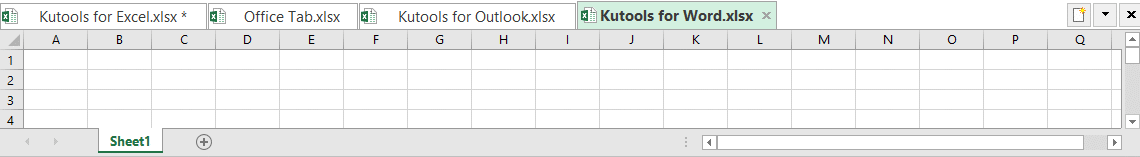## Excel random data: generate random numbers, texts, dates, times in Excel

Generally speaking, random data are a series of numbers, text strings or other symbols which are used in statistical sampling, data encryption, lottery, testing or training, or other fields where unpredictable results are desired. In this article, we will introduce different methods for generating random numbers, text strings, dates and times in normal Excel and Excel 365.

### Generate random numbers, texts, dates, times in Excel

This section will talk about various kinds of solutions for generating numbers, text strings, dates and times in an Excel worksheet.

#### 1.1 Generate random numbers in Excel

To generate or insert multiple random numbers in a worksheet, the normal RAND or RANDBETWEEN function can help you a lot. Besides the formulas, there are other codes and easy tools that also can do you a favor.

###### RAND function to generate random numbers

Generate random decimal numbers between two numbers

The RAND function can be used to generate random decimal numbers between 0 and 1, between 0 and any other number or between two specific numbers.

 Formula Description =RAND() Generate random decimal numbers between 0 and 1. =RAND()*N Generate random decimal numbers between 0 and N. =RAND()*(B-A)+A Generate random decimal numbers between any two numbers you specified. (A is the lower bound value and B is the upper bound value.)

Please copy the above formula you need, and apply the formula to as many cells as you want, then you will get the results as below screenshots shown:

 =RAND() =RAND()*50 =RAND()*(100-50)+50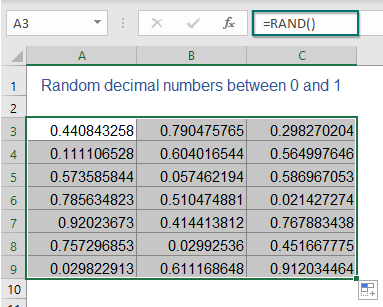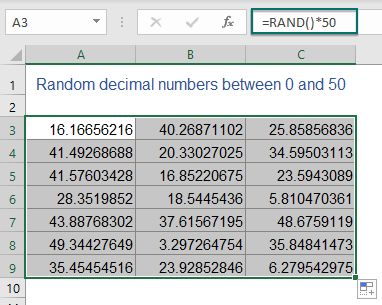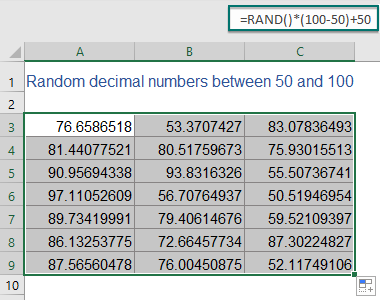Generate random integer numbers between two numbers

To generate some random integers, you should combine the RNAD and INT functions together as below table shown:

 Formula Description =INT(RAND()*N) Generate random integers between 0 and N. =INT(RAND()*(B-A)+A) Generate random integers between any two numbers you specified. (A is the lower bound value and B is the upper bound value.)

Please apply the above formula you need, then drag and copy the formula to other cells as you need, and then you will get the results as below screenshots shown:

 =INT(RAND()*100) =INT(RAND()*(500-200)+200)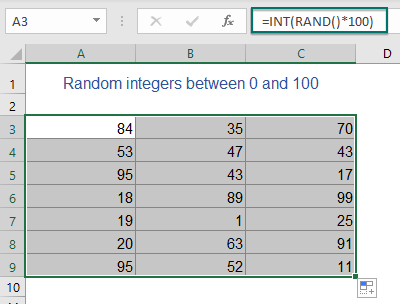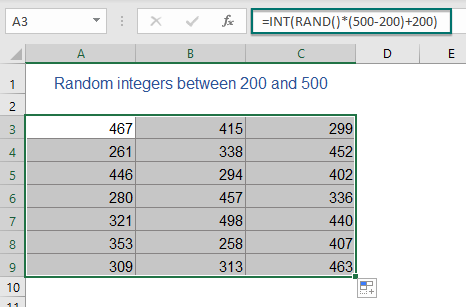###### RANDBETWEEN function to generate random numbers

In Excel, there is a RNDBETWEEN function which also can help you to create random numbers quickly and easily.

Generate random integer numbers between two numbers

=RANDBETWEEN(bottom, top)
• bottom, top: The lowest and highest numbers of the random numbers range you want to get.

For example, if you want to generate random integers between 100 and 200, please apply the below formula into a blank cell, and then drag and copy the formula to other cells you want, see screenshot:

=RANDBETWEEN(100, 200)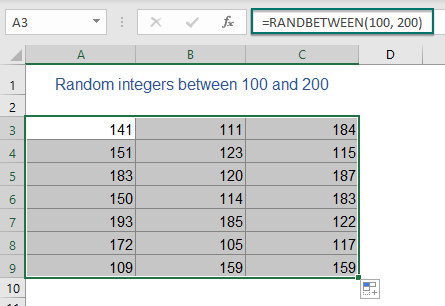Tips: This RANDBETWEEN function also can create both positive and negative numbers. To insert random numbers between -100 and 100, you just need to change the bottom value to -100, see below formula:

=RANDBETWEEN(-100, 100)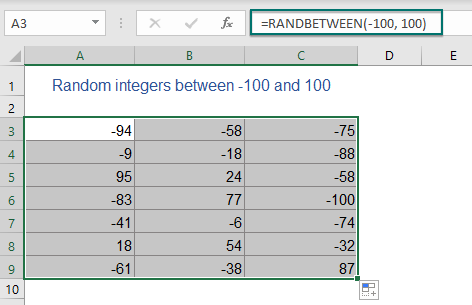Generate random numbers with specified decimal places between two numbers

To create random numbers with specified decimal places, you need to change the RANDBETWEEN formula as this:

• Random numbers with one decimal place: =RANDBETWEEN(bottom*10, top*10)/10
• Random numbers with two decimal places: =RANDBETWEEN(bottom*100, top*100)/100
• Random numbers with three decimal places: =RANDBETWEEN(bottom*1000, top*1000)/1000
• ...

Here, I want to get a list of random numbers between 10 and 50 with two decimal places, please apply the below formula, and then drag and copy the formula to other cells as you need, see screenshot:

=RANDBETWEEN(10*100, 50*100)/100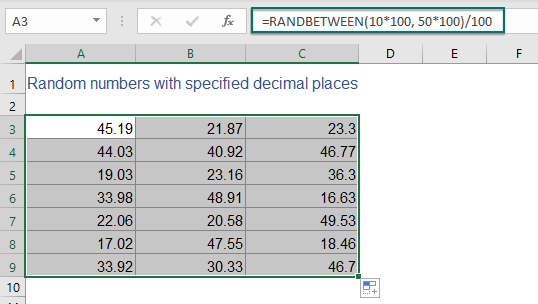###### User Defined Function to generate random numbers between two values

The following User Defined Function also can help you to generate random integer numbers or numbers with specific decimal places in a range of worksheet. Please do as follows:

1. Hold down ALT + F11 keys to open the Microsoft Visual Basic for Applications window.

2. Click Insert > Module, and paste the following code in the Module window.

VBA code: Generate random numbers

``````Public Function RandomNumbers(Num1 As Long, Num2 As Long, Optional Decimals As Integer)
'Updateby Extendoffice
Application.Volatile
Randomize
If IsMissing(Decimals) Or Decimals = 0 Then
RandomNumbers = Int((Num2 + 1 - Num1) * Rnd + Num1)
Else
RandomNumbers = Round((Num2 - Num1) * Rnd + Num1, Decimals)
End If
End Function
``````

3. Then, close the code and go back to the worksheet, in a blank cell, type this formula =RandomNumbers(X,Y,Z).

Note: In the above formula, X indicates the lower limit of the numbers, Y indicates the upper limit of the numbers, and Z is the specified decimal places of random numbers, please change them to your needed numbers.

1.) To generate random whole numbers between 50 and 200, please use this formula:

=RandomNumbers(50,200,0)

2.) To insert random numbers between 50 and 200 with 2 decimal places, please apply the below formula:

=RandomNumbers(50,200,2)

4. At last, drag and copy the formula to other cells as you want, you will get the results as below screenshots shown: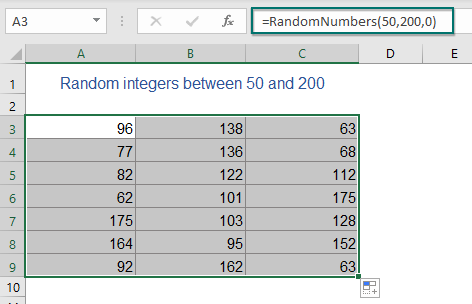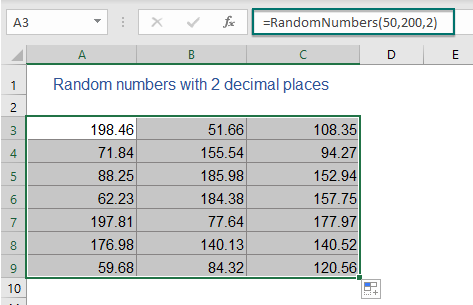###### A handy feature to generate random numbers between two numbers

If you are tired with remembering and entering formulas, here, I will recommend a useful feature – Insert Random Data of Kutools for Excel. With this feature, you can generate random integers or decimal numbers without any formulas.

After installing Kutools for Excel, please do as this:

1. Select a range of cells where you want to generate random numbers, and then click Kutools > Insert > Insert Random Data, see screenshot: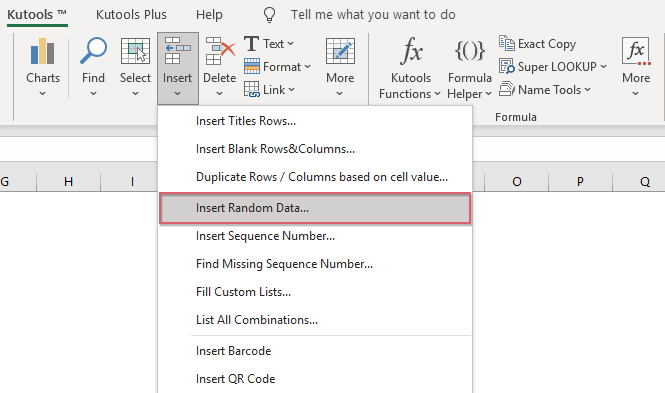2. In the Insert Random Data dialog box:

1.) Generate random whole numbers:

Under the Integer tab, in the From and To boxes, type the number range you will generate random whole numbers between, and then click Ok or Apply button to get the random whole numbers as below screenshot shown: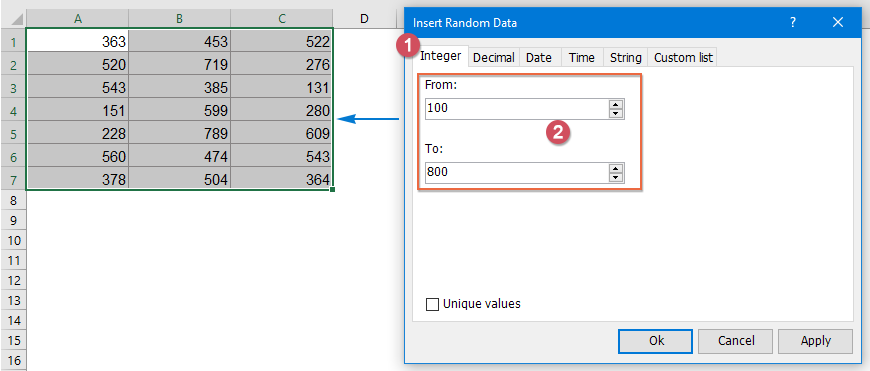2.) Generate random numbers with specific decimal places:

Under the Decimal tab, specify two numbers separately in the From and To boxes that you want to generate random decimal numbers between. And then choose decimal place in Decimal place text box and click the Ok or Apply button to generate random decimals. See screenshot: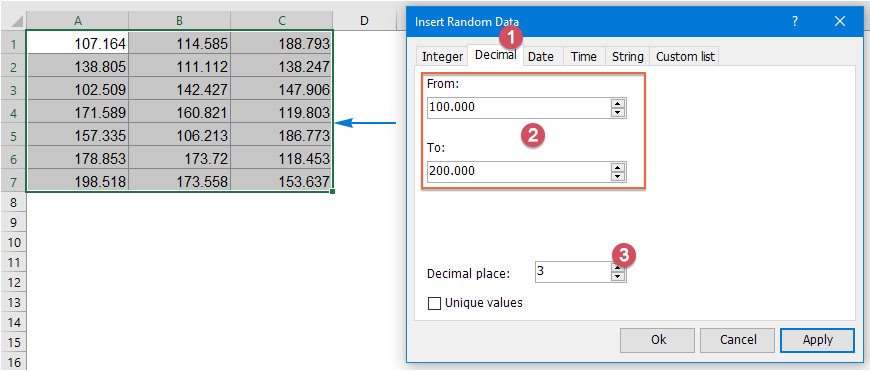Tips: To generate random decimals with no duplicates, please check the Unique values option.

#### 1.2 Generate random numbers without duplicates (unique random numbers)

When using the formulas or code to generate random numbers, some duplicate numbers will be produced as well. If you want to create a list of random numbers without duplicates, this section will demonstrate some methods for you.

###### Generate random numbers without duplicates with an array formula

For instance, I want to generate random numbers between 100 to 200 without duplicate numbers, here is a complex array formula that could help you, please do with the following steps:

1. Specify lower limit and upper limit values in two cells. In this example , I will enter 100 and 200 into cell B2 and B3, see screenshot: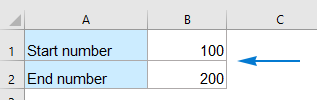2. Then, copy the following formula into a blank cell, D3 for example, (don’t put the formula into a cell of the first row), and then press Ctrl+ Shift + Enter keys together to get the first numbers, see screenshot:

=IFERROR(LARGE(ROW(INDIRECT(\$B\$1&":"&\$B\$2))*NOT(COUNTIF(\$D\$2:D2,ROW(INDIRECT(\$B\$1&":"&\$B\$2)))),RANDBETWEEN(1,\$B\$2-\$B\$1-ROW(A1)+2)),"")

Note: In the above formula, B1 is the lower value, and B2 is the upper value you want to return random numbers between. D2 is the cell above the formula.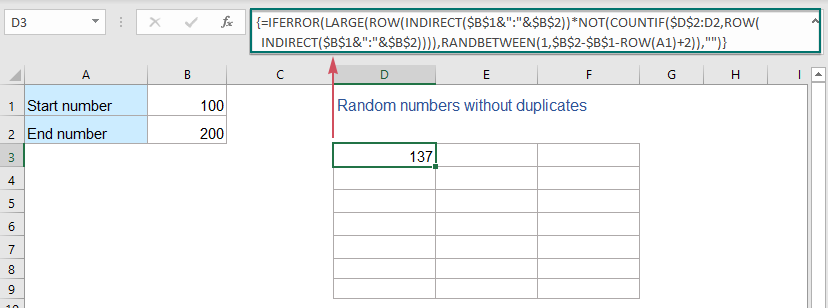3. Then, drag and copy this formula to other cells as you want to generate the random numbers between 100 and 200: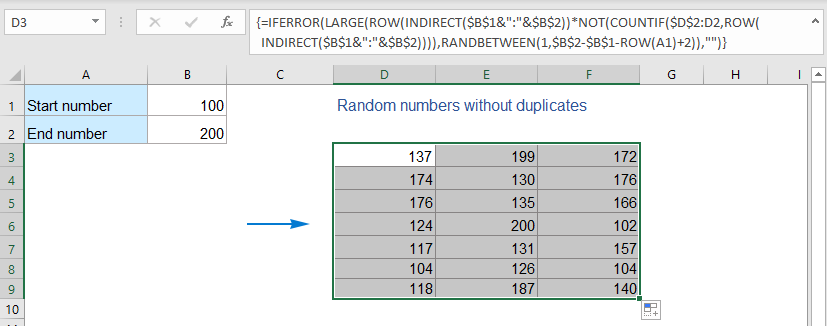###### Generate random numbers without duplicates with VBA code

If the above formula is somewhat difficult for you to understand, you can apply the below VBA code, please do as this:

1. Hold down ALT + F11 keys to open the Microsoft Visual Basic for Applications window.

2. Click Insert > Module, and paste the following code in the Module window.

VBA code: Generate random numbers without duplicates

``````Sub Range_RandomNumber()
'Updateby Extendoffice
Dim xStrRange As String
Dim xRg, xCell, xRg1 As Range
Dim xArs As Areas
Dim xNum_Lowerbound As Integer
Dim xNum_Upperbound  As Integer
Dim xI, xJ, xS, xR As Integer
xStrRange = "A1:B20"
xNum_Lowerbound = 100
xNum_Upperbound = 200
Set xRg = Range(xStrRange)
Set xArs = xRg.Areas
xRgCount = 0
For xI = 1 To xArs.Count
Set xCell = xArs.Item(xI)
xRgCount = xCell.Count + xRgCount
Next xI
xS = (xNum_Upperbound - xNum_Lowerbound + 1)
If xRgCount > xS Then
MsgBox ("Number of cells greater than the number of unique random numbers!")
Exit Sub
End If
xRg.Clear
For xI = 1 To xArs.Count
Set xCell = xArs.Item(xI)
For xJ = 1 To xCell.Count
Set xRg1 = xCell.Item(xJ)
xR = Int(xS * Rnd + xNum_Lowerbound)
Do While Application.WorksheetFunction.CountIf(xRg, xR) >= 1
xR = Int(xS * Rnd + xNum_Lowerbound)
Loop
xRg1.Value = xR
Next
Next
End Sub
``````

Note: In the above code, xStrRange = "A1:B20" indicates that you want to generate random numbers in the range A1:B20. xNum_Lowerbound = 100 and xNum_Upperbound = 200 indicate that the lower and upper values to create random numbers between 100 and 200. Please change them to your need.

3. Then press F5 key to run this code, and the unique random numbers will be inserted into the specified range.

###### Generate random numbers without duplicates with a powerful feature

To quickly and create multiple unique random numbers, the Kutools for Excel’s Insert Random Data feature supports a smart option – Unique values. By checking this small option, you will solve this task with ease.

After installing Kutools for Excel, please do as this:

1. Select a range of cells where you want to generate random numbers.

2. And then click Kutools > Insert > Insert Random Data. In the popped-out dialog box, please do the below operations:

• Under the Integer tab, in the From and To boxes, type the number range you will generate random numbers between;
• Check the Unique values option;
• Then click Ok or Apply button to get the unique random numbers as below screenshot shown.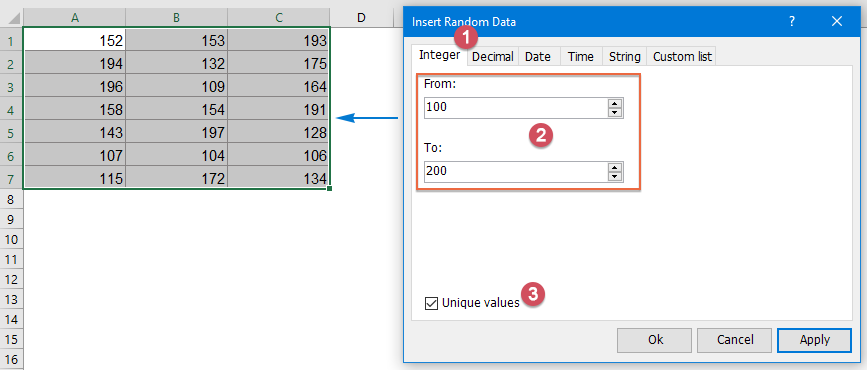#### 1.3 Generate random even or odd numbers in Excel

If you want to generate some random even or odd numbers in a range of cell, you just need to put the RANDBETWEE function inside the EVEN or ODD function, the generic syntaxes are:

 Formula Description =EVEN(RANDBETWEEN(bottom,top)) Generate random even numbers between two given numbers. =ODD(RANDBETWEEN(bottom,top)) Generate random odd numbers between two given numbers.

For instance, to generate random even or odd numbers from 10 to 100, please apply the following formulas:

=EVEN(RANDBETWEEN(10,100))             (Generate random even numbers)
=ODD(RANDBETWEEN(10,100))
(Generate random odd numbers)

And then, copy the formula to other cells you want by dragging the fill handle, then, you will get the results as below screenshots shown: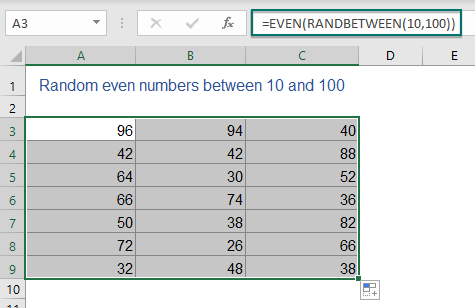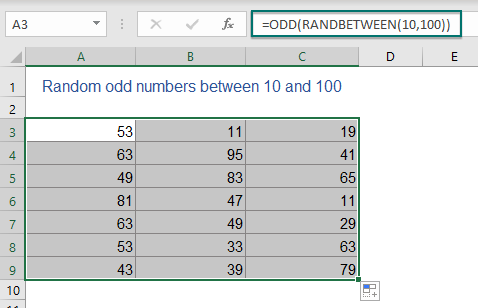#### 1.4 Generate random numbers that add up to a specified value

Sometimes, you may need to create a set of random numbers that add up to a predetermined value. For example, I want to generate 5 or n random numbers between 10 and 50 that come to a total of 100 as below screenshot shown. To solve this puzzle in Excel, I will introduce two methods for you.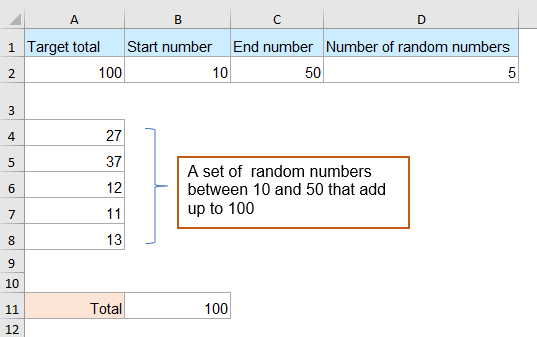###### Generate random numbers that add up to a specific value with formulas

1. First, you should create your needed data: the predetermined total value, start number, end number and how many random numbers you want to generate as below screenshot shown: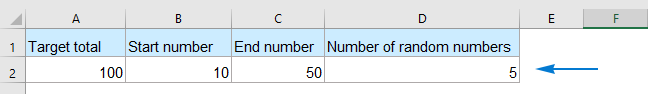2. Then, please copy the below formula into a blank cell where you want to generate the numbers. In this example, I will put the formula into cell A4, and press Enter key to get the first random number, see screenshot:

=RANDBETWEEN(MAX(\$B\$2,\$A\$2-((\$D\$2-ROWS(\$A\$4:\$A4))*\$C\$2)),MIN(\$C\$2,\$A\$2-((\$D\$2-ROWS(\$A\$4:\$A4))*\$B\$2)))

Note: In the above formula: A2 is the given total value; B2 and C2 are bottom and top values you want to generate random numbers between; D2 indicates the number of random numbers you want to generate; A4 is the cell where you enter this formula.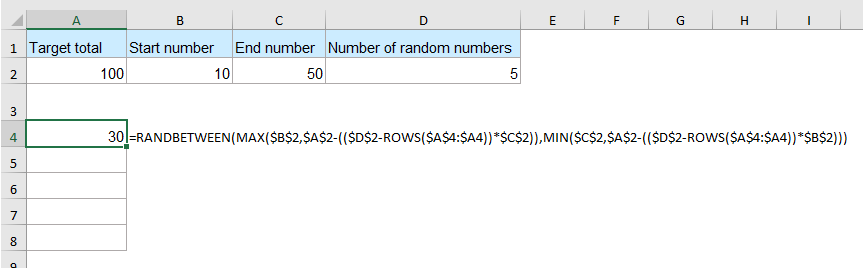3. Go on copying the following formula into cell A5, and press Enter key to get the second random number, see screenshot:

=IF(ROW()=\$D\$2+3,\$A\$2-SUM(\$A\$4:\$A4),IF(ROW()>\$D\$2+3,"",RANDBETWEEN(MAX(\$B\$2,\$A\$2-(SUM(\$A\$4:\$A4)+(\$D\$2-ROWS(\$A\$4:\$A5))*\$C\$2)),MIN(\$C\$2,\$A\$2-(SUM(\$A\$4:\$A4)+(\$D\$2-ROWS(\$A\$4:\$A5))*\$B\$2)))))

Note: In the above formula: A2 is the given total value; B2 and C2 are bottom and top values you want to generate random numbers between; D2 indicates the number of random numbers you want to generate; A4 is the cell to put the first formula; and A5 is the cell to put the second formula.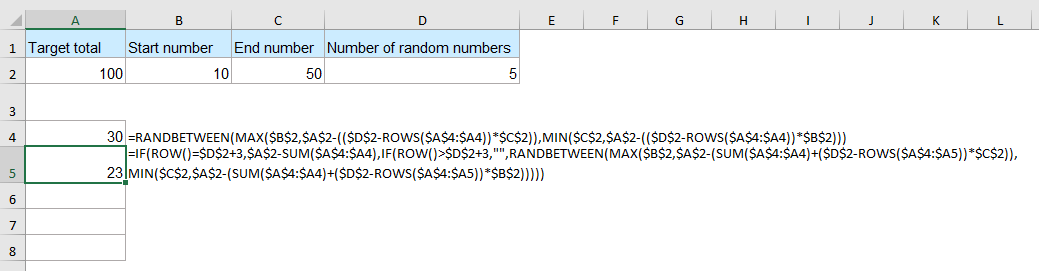4. Then, select the second generated number, drag down to copy this formula to the below three cells. And now, you will get 5 random numbers as below screenshot shown: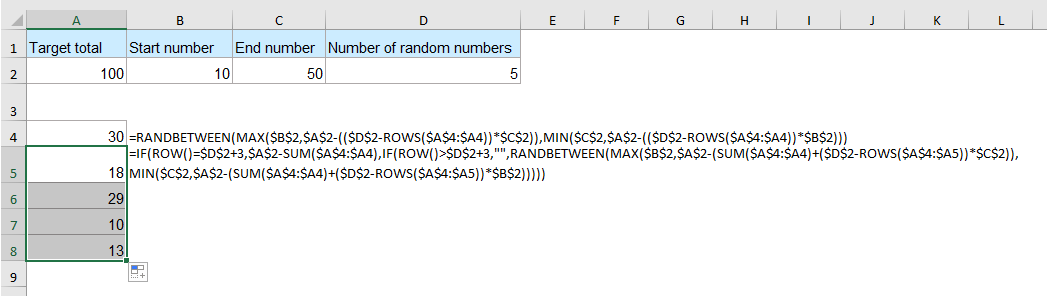5. To test the result, you can sum these numbers to check if the total is 100, and you can press F9 to refresh the random numbers dynamically, and their total sum is always 100.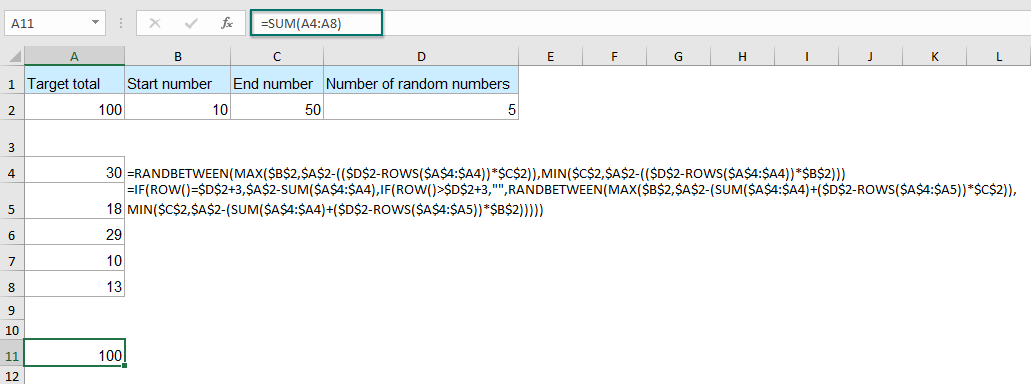###### Generate random number combinations that add up to a specific value with an amazing feature

We can use the above formulas to generate random numbers that meet our needs. However, if you want to list all the possible number combinations that consist of the numbers you specified with a specific total sum, here, I will recommend an easy tool – Kutools for Excel. With its Make Up A Number feature, you can get all sets of random number combinations with the same specific sum.

After installing Kutools for Excel, please do as this:

1. First, you should list the numbers you specified. Here, we listed all the numbers between 10 and 50 as below screenshot shown: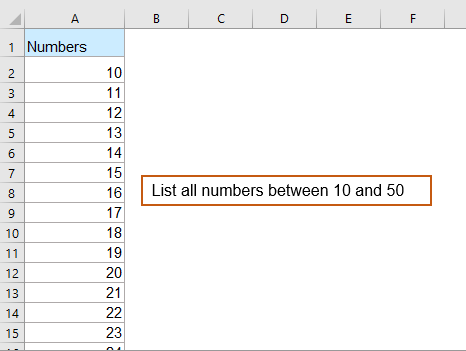2. Then, click Kutools > Content > Make Up A Number, see screenshot: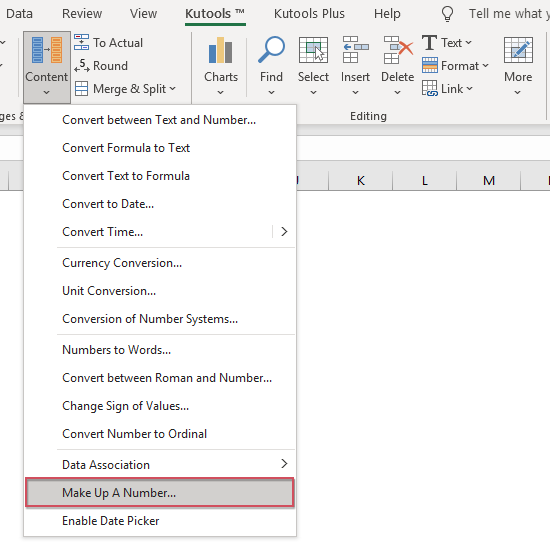3. In the popped-out Make up a number dialog box, please do the following operations:

• In the Data Source box, select the number list to find which numbers add up to 100;
• Under the Options, enter the total value in the Sum text box. Here we typed 100 into the text box;
• Check Save in a new sheet option if you want to list the results in a new sheet;
• Click the OK button. See screenshot: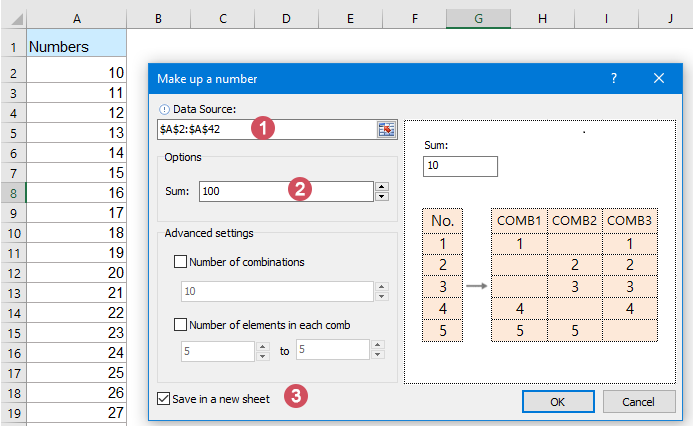4. After processing, you will see all sets of random numbers with a total sum of 100 that consist of the number from 10 to 50 are listed as follows.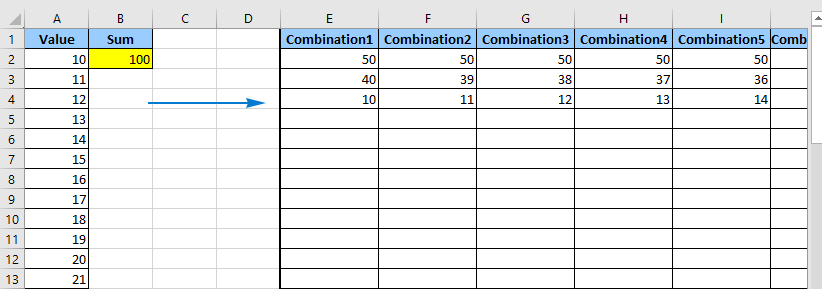Tips: It is optional for you to specify the number of combinations and number of random numbers in each combination. For example, to generate 10 combinations and each combination contains 5 random numbers, you can set the operations in the dialog box under Advanced settings as follows: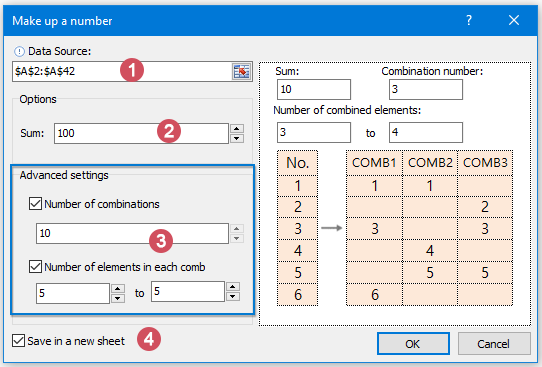And you will get the results as this: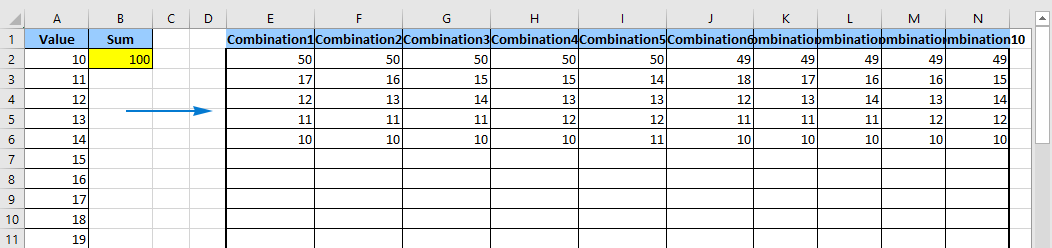#### 1.5 Generate random letters and text strings with formulas

This section will show you how to generate random letters in Excel, such as uppercase letters from A to Z, lowercase letters from a to z or some special character (! " # \$ % & ' ( ) * + , - . /).

###### Generate random letters and text strings with formulas

In Excel, you can combine the CHAR and RANDBETWEEN functions with some ANSI character codes to create some formulas as shown below:

 Formula Description =CHAR(RANDBETWEEN(65, 90)) Generate random uppercase letters between A and Z. =CHAR(RANDBETWEEN(97, 122)) Generate random lowercase letters between a and z. =CHAR(RANDBETWEEN(33, 47)) Generate random special characters, such as: ! " # \$ % & ' ( ) * + , - . /

Please apply any one of the above formulas you need, and copy the formula into as many cells as you want, then you will get the results as below screenshots shown:

 =CHAR(RANDBETWEEN(65, 90)) =CHAR(RANDBETWEEN(97, 122)) =CHAR(RANDBETWEEN(33, 47))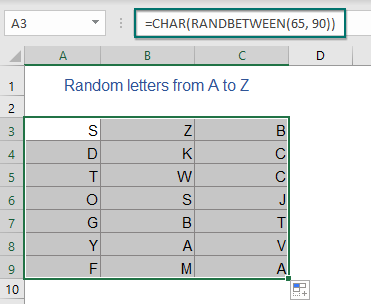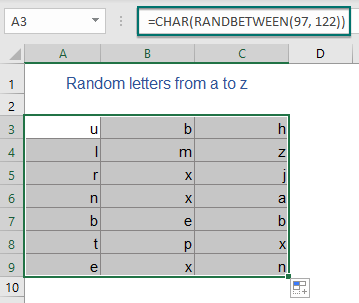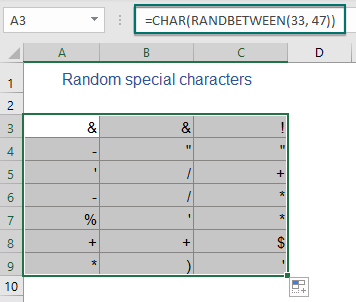Tips: If you want to generate random text strings with several letters, you just need to use the & character to join the letters as you need.

1.) To generate random strings with four uppercase letters, please apply the below formula:

=CHAR(RANDBETWEEN(65,90))& CHAR(RANDBETWEEN(65,90)) & CHAR(RANDBETWEEN(65,90)) & CHAR(RANDBETWEEN(65,90))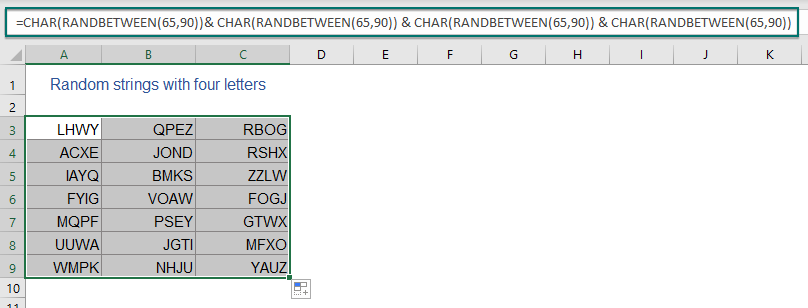2.) To generate random strings with four lowercase letters, please apply the below formula:

=CHAR(RANDBETWEEN(97,122))& CHAR(RANDBETWEEN(97,122)) & CHAR(RANDBETWEEN(97,122)) & CHAR(RANDBETWEEN(97,122))

3.) To generate random strings with the first two uppercase letters and the last two lowercase letters, please use the following formula:

=CHAR(RANDBETWEEN(65,90))& CHAR(RANDBETWEEN(65,90)) & CHAR(RANDBETWEEN(97,122)) & CHAR(RANDBETWEEN(97,122))

You can use the simple formulas and & character to make various combinations that meet your needs.

###### Generate random letters and text strings with a handy feature

If you have installed Kutools for Excel, you can generate random letters and strings quickly and easily without remembering any formulas.

After installing Kutools for Excel, please do as this:

1. Select a range of cells to insert the letters or strings.

2. And then click Kutools > Insert > Insert Random Data, in the popped-out dialog box, please do the following operations:

• Click the String tab;
• Check a-z or A-Z or both of them that you want to insert;
• Then, specify the length of the string you want in the String length text box;
• At last, click Ok or Apply to insert the strings as below screenshot shown.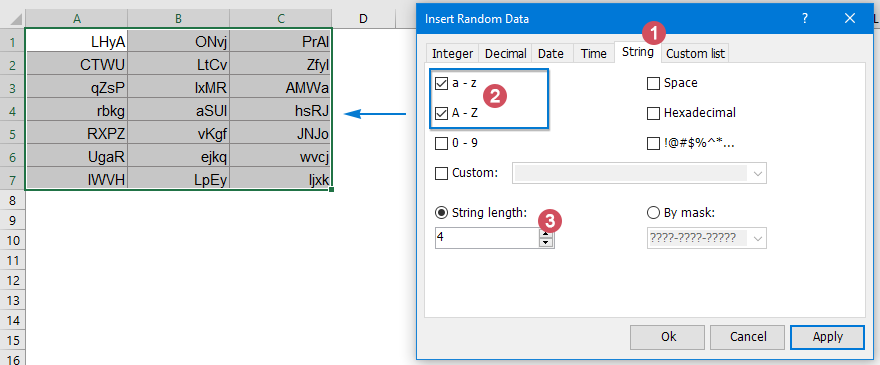#### 1.6 Generate random passwords with alphanumeric characters in Excel

When you create a password, the password should have 8 characters at least and contain a mix of uppercase letters, lowercase letters, numbers and some special characters. In this section, I will introduce some tricks for generating random passwords in Excel.

###### Generate random passwords with alphanumeric characters by using formulas

For instance, here, I will create random passwords with the length of 8 characters. You just need to combine the three formulas that are provided in Generate random letters and text strings with formulas section.

Please copy the below formula into a blank cell:

=CHAR(RANDBETWEEN(65,90))&CHAR(RANDBETWEEN(97,122))&CHAR(RANDBETWEEN(97,122))&CHAR(RANDBETWEEN(65,90))&RANDBETWEEN(100,999)&CHAR(RANDBETWEEN(33,47))

Note: In the above formula, the first CHAR and RANDBETWEEN functions will generate a random uppercase letter, the second and third expression will generate two lowercase letters, the fourth expression is used to generate one uppercase letter, the fifth expression generates a 3-digit number between 100 and 999, and the last expression is used to generate a special character, you can modify or adjust the order of them to your need.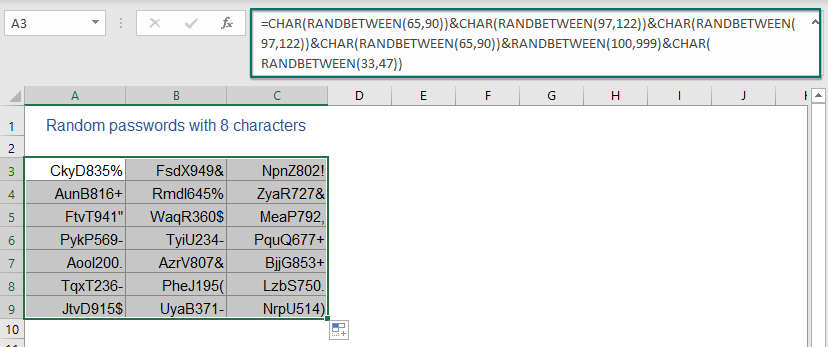###### Generate random passwords with alphanumeric characters by using User Defined Function

To insert random passwords in Excel, the following User Defined Function also can do you a favor, please do as this:

1. Hold down the ALT + F11 keys, and it opens the Microsoft Visual Basic for Applications window.

2. Click Insert > Module, and paste the following macro in the Module window.

VBA code: Generate random passwords in Excel

``````Function RandomizeF(Num1 As Integer, Num2 As Integer)
'Updateby Extendoffice
Dim Rand As String
Application.Volatile
getLen = Int((Num2 + 1 - Num1) * Rnd + Num1)
Do
i = i + 1
Randomize
Rand = Rand & Chr(Int((85) * Rnd + 38))
Loop Until i = getLen
RandomizeF = Rand
End Function
``````

3. Then close the code and go back to the worksheet. In a cell, enter this formula =RandomizeF(8,10) to generate a random text string with a minimum length of 8 characters, and a maximum length of 10 characters.

4. Then drag and copy the formula to other cells as you want. The random strings with alphanumeric and specific characters with the length of between 8 and 10 will be created. See screenshot: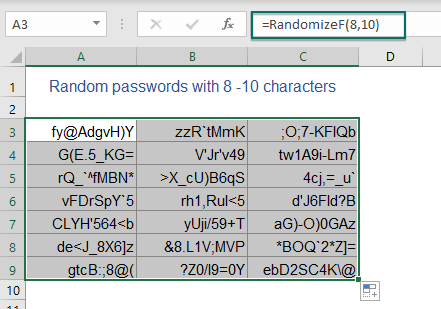###### Generate random passwords with alphanumeric characters by using an easy feature

Is there any quick and easy way to generate multiple random passwords in excel? Kutools for Excel provides an excellent feature - Insert Random Data. With this feature, you can insert random passwords with only several clicks.

After installing Kutools for Excel, please do as this:

1. Select a range of cells where to insert the passwords.

2. Then click Kutools > Insert > Insert Random Data. In the popped-out dialog box, please do the following operations:

• Click the String tab;
• Check the type of characters as you need;
• Then, specify the length of the password you want in the String length text box;
• At last, click Ok or Apply to generate the passwords as below screenshot shown.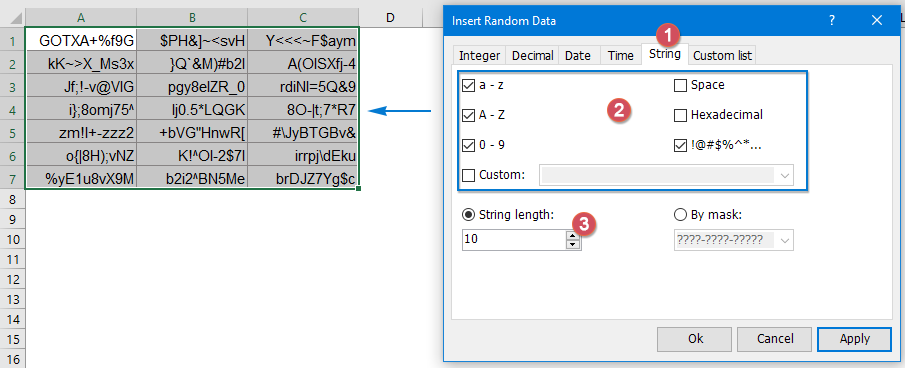#### 1.7 Generate random specific texts in Excel

Have you ever tried to display or list some specific text values randomly in Excel? For example, to list some given texts (item1, itme2, item3, item4, item5) randomly in a list of cells, the following two tricks may help you solve this task.

###### Generate random specific texts with a formula

In Excel, you can create a formula based on the CHOOSE and RANDBETWEEN functions to randomly list the specific texts, the generic syntax is:

=CHOOSE(RANDBETWEEN(1,n),"Value_1","Value_2","Value_3",…"Value_n")
• Value_1, Value_2, Value_3, Value_n : Represent the text values you want to list randomly;
• n : The number of the text values you want to use.

Please apply the below formula into a blank cell, and then drag the fill handle down to fill the cells where you want to list the specific values randomly, see screenshot:

=CHOOSE(RANDBETWEEN(1,5),"Chemistry","Physics","Geography","Biology","Economics")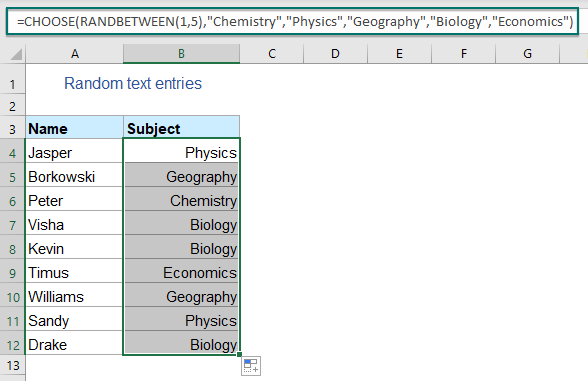###### Generate random specific texts with a quick method

If you have Kutools for Excel, its Insert Random Data feature also can help you to insert custom text values randomly in a range of cells.

After installing Kutools for Excel, please do as this:

1. Select a range of cells where to insert the specific texts.

2. Then click Kutools > Insert > Insert Random Data, in the popped-out dialog box, please do the following operations:

• Click the Custom list tab;
• Then, click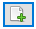button to open another Kutools for Excel prompt box, enter or select your own custom text values that you want to list randomly. (The entries should be separated by comma when typing them manually.)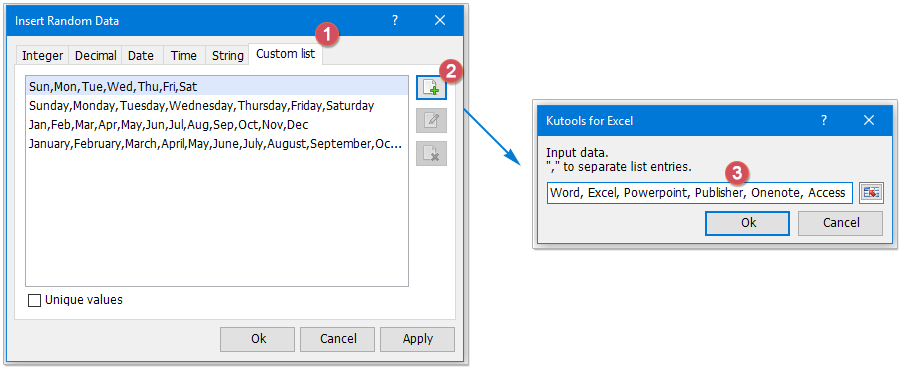3. Then, click Ok to return to the Insert Random Data dialog, your own custom texts list has been displayed into the list box. Now, select the new list items, click Ok or Apply button to insert the values into selected cells randomly.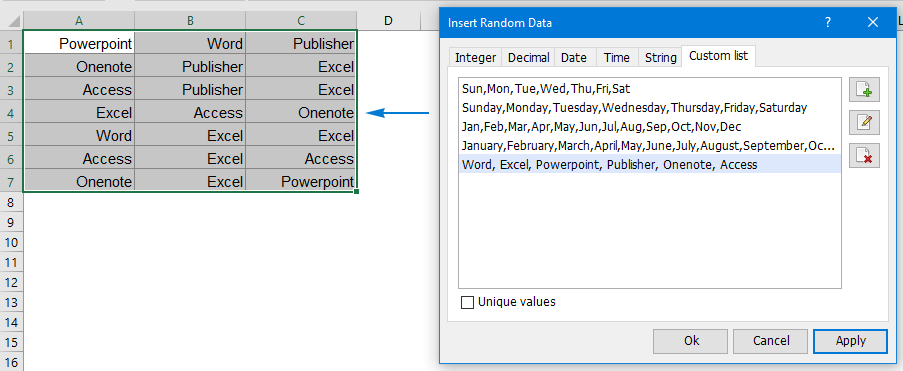Tips: To list randomly the specified texts without duplicates, please check Unique values option.

#### 1.8 Generate or select random values from a list in Excel

Supposing, you have a long list of names, to pick up some random names from that list as the lucky names or research objects as below screenshot shown. How could you solve this problem in excel?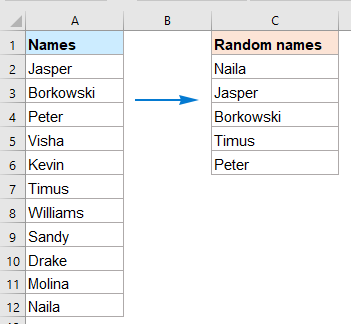###### Generate random values from a list with INDEX, RANDBETWEEN and ROWS functions

In Excel, there is no direct way for us to pull out random values from a list, but you can create a formula based on the INDEX, RANDBETWEEN and ROWS functions to extract some random values.

1. Please copy the below formula into a blank cell where you want to put the extracted value:

=INDEX(\$A\$2:\$A\$12,RANDBETWEEN(1,ROWS(\$A\$2:\$A\$12)),1)

Note: In the above formula, A2:A12 is the list of values that you want to pick up random values from.

2. Then, drag the fill handle down to several cells where you want to display the random values, and you will get the result as following screenshot shown: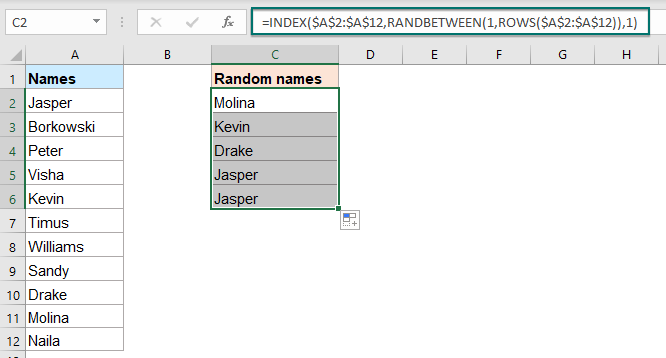###### Generate random values from a list without duplicates with INDEX, RANK.EQ functions

When using the above formula, some duplicate values will be displayed. To skip the duplicate values, you should create a helper column first, and then apply a formula based on the INDEX and RANK.EQ functions. Please do as follows:

1. Enter the below formula into a blank cell to get a list of random numbers, see screenshot:

=RAND()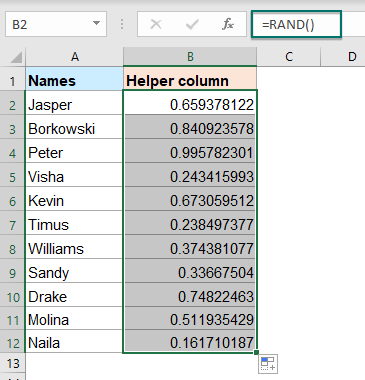2. Then, copy the below formula in a cell of another column where you want to extract some random values, and then drag and copy this formula to the below cells to display some non-repeating random values, see screenshot:

=INDEX(\$A\$2:\$A\$12,RANK.EQ(\$B2,\$B\$2:\$B\$12))

Note: In the above formula, A2:A12 is the list of values you want to generate some random values from, B2 is the first cell of the helper column, B2:B12 is the helper formula cells you are created in step 1.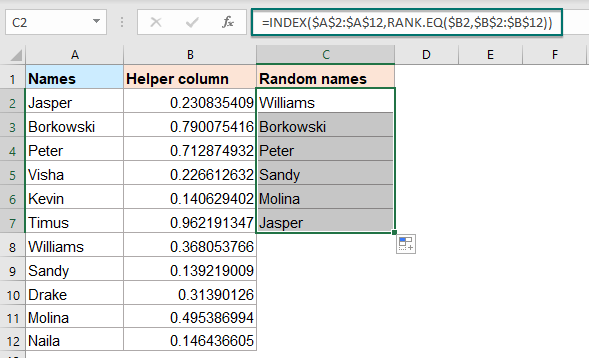###### Select random cells, rows, columns from a range with an awesome feature

Here I will recommend a useful feature – Sort / Select Range Randomly of Kutools for Excel. With this feature, you can select some random cells, rows or columns as you need.

After installing Kutools for Excel, please do as this:

1. Select the list of cells you want to select some random values from.

2. And then, click Kutools > Range > Sort / Select Range Randomly, see screenshot: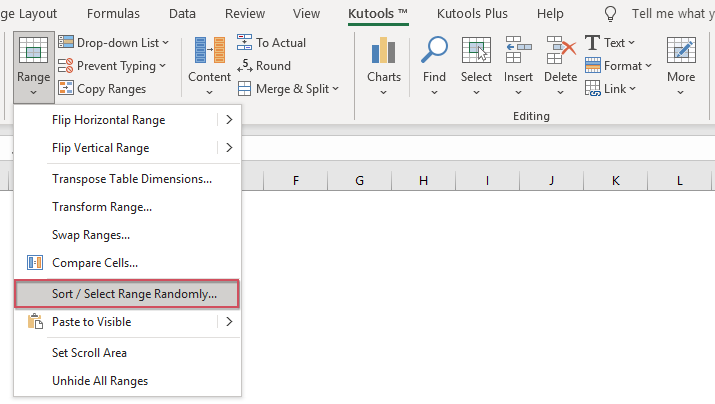3. In the Sort / Select Range Randomly dialog box, please do the following operations:

• Click Select tab;
• Then, enter the number of cells you want to select randomly in the No. of cells to select box;
• In the Select Type section, choose one operation as you want. In this case, I will choose Select random cells option.
• And then, click Ok or Apply button, five cells will be selected randomly at once, see screenshots: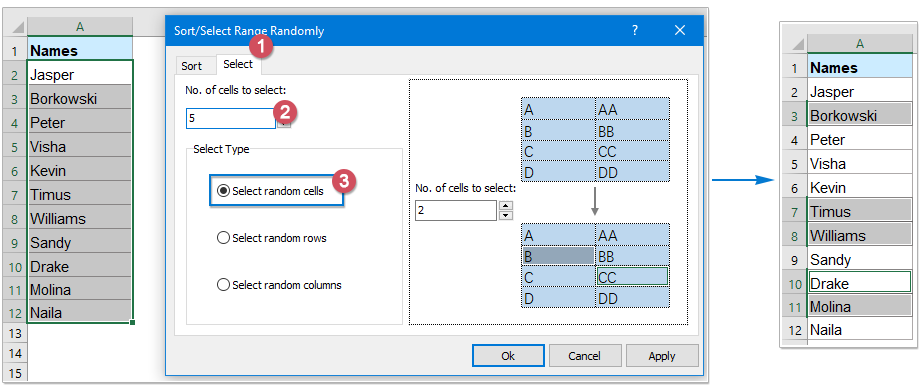4. After selecting the cells, you can copy and paste them to other cells as you need.

#### 1.9 Assign data to groups randomly in Excel

Assume that you have a list of names, now, you want to divide the names to three groups (Group A, Group B, Group C) randomly as below screenshot shown. In this section, I will discuss some formulas for solving this task in Excel.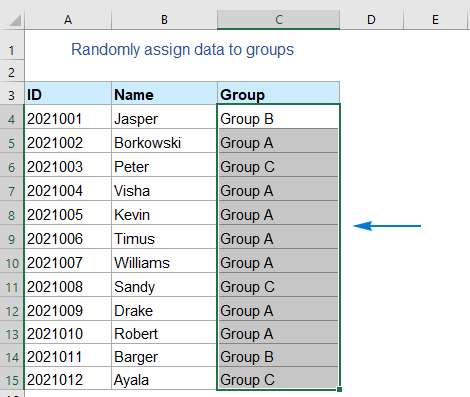###### Assign data to group randomly with a formula

To randomly assign people to specified groups , you can use the CHOOSE function in combination with the RANDBETWEEN function.

1. Please copy or enter the following formula into a cell where you want to generate the group:

=CHOOSE(RANDBETWEEN(1,3),"Group A","Group B","Group C")

Note: In the above formula, Group A, Group B, and Group C indicate the group names you want to assign, and the number 3 indicates how many groups you want to distribute.

2. Then, drag the fill handle to fill this formula to other cells, and the names will be divided into three groups as below screenshot shown: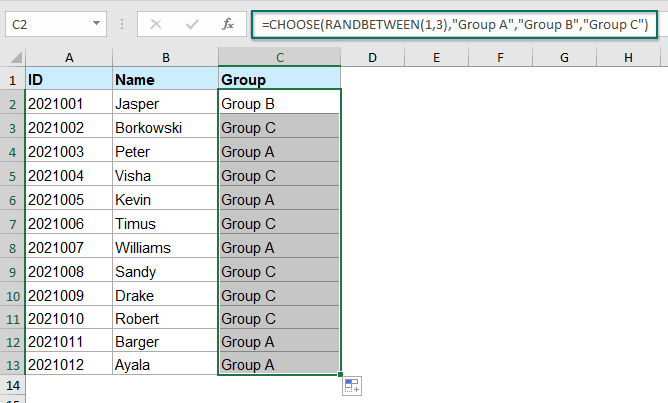###### Assign data to group with equal number randomly with a formula

If you want all the groups have an equal number of names, the above formula will not work correctly for you. In this case, you can create a helper column with random values by the RAND function, and then apply a formula based on the INDEX, RANK and ROUNDUP functions.

For instance, I list the group names that you want to assign based on in the cells F2:F4. To assign people to the groups (Group A, Group B, Group C), and each group has 4 participants, please do as this:

1. Enter this formula: =RAND() into a blank cell to get a list of random numbers, see screenshot: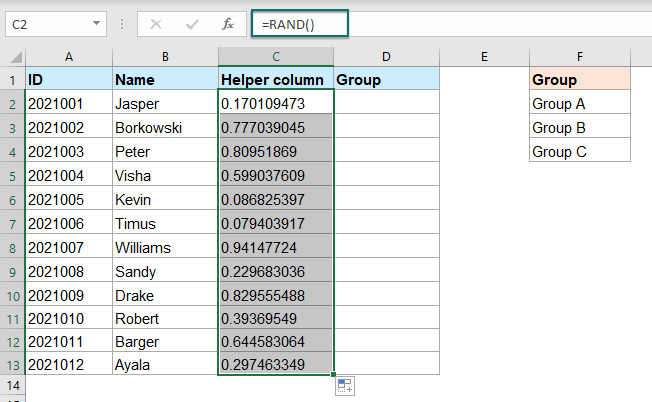2. Then, In the next column, for instance, in cell D2, copy or type the below formula:

=INDEX(\$F\$2:\$F\$4, ROUNDUP(RANK(C2,\$C\$2:\$C\$13)/4,0))

Note: In the above formula, C2 is the first cell of the helper column, C2:C13 is the helper formula cells you created in step 1, the number 4 indicates how many names you want each group contains, F2:F4 is the range of cells contain the group names that you want to assign for the data.

3. Drag fill handle down to generate random groups for the list of data, and the names will be divided into equal groups, see screenshot: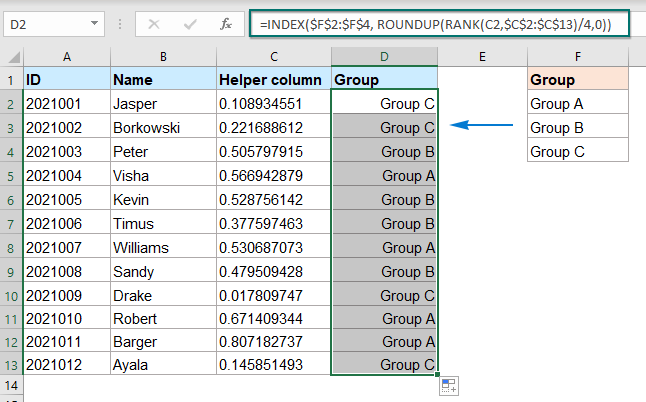#### 1.10 Generate random dates in Excel

To produce some arbitrary dates between two given dates, here, I will introduce some methods for you.

###### Generate random dates between two given dates with formulas

For example, I want to generate some dates between 2021-5-1 and 2021-10-15 randomly. Normally, in Excel, you can accomplish the task by using a combination of the RANDBETWEEN and DATE functions, please do as this:

1. Select a cell where you want to insert a random date, and then enter the following formula:

=RANDBETWEEN(DATE(2021, 5, 1),DATE(2021, 10, 15))

Note: In this formula, 2021, 5, 1 is the starting date, and 2021, 10, 15 is the ending date, you can replace them as you need.

2. Then, drag and copy this formula to other cells that you want to fill this formula, five-digit numbers will be displayed in the cells as below screenshot shown: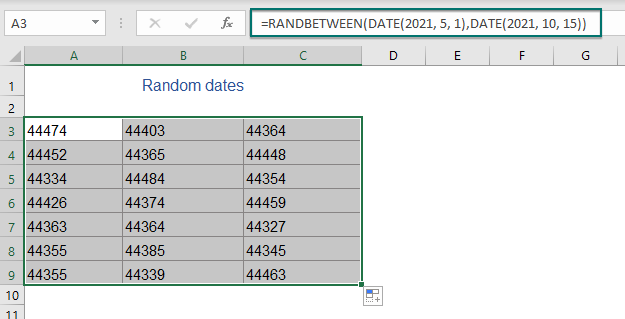3. And then, you should format the numbers to date format. Please select the formula cells, and right-click, choose Format Cells from the context menu.

4. In the Format Cells dialog box, click Number tab, and select Date from the Category pane, then select a date format you need from the Type drop-down list. See screenshot: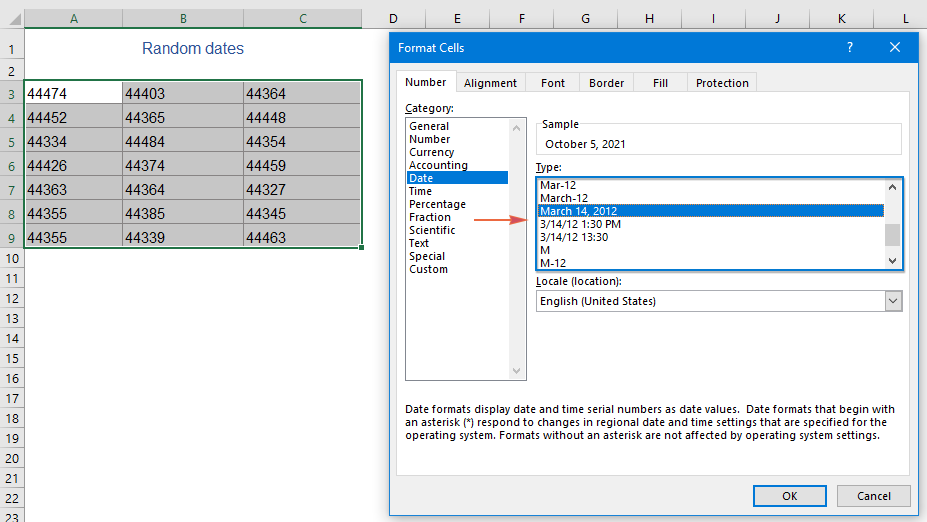5. Click OK to close the dialog. Now, the numbers have been converted to the normal dates. See screenshot: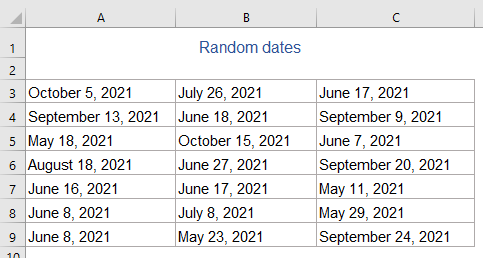Tips: If you want to generate random workdays excluding the weekends, the below formula may help you:

=WORKDAY(RANDBETWEEN(DATE(2021, 5, 1),DATE(2021, 10, 15))-1,1)

###### Generate random dates between two given dates with an amazing feature

Kutools for Excel’s Insert Random data also provides an option to help you to generate random dates, workdays, weekends between two given dates.

After installing Kutools for Excel, please do as this:

1. Select a range of cells where to insert the random dates.

2. Then click Kutools > Insert > Insert Random Data, in the popped-out dialog box, please do the following operations:

• Click the Date tab;
• Then, specify the date scope. In this example, I will choose from 5/1/2021 to 10/15/2021.
• And then, choose the date type - workday date, weekend date or both of them as you need.
• At last, click Ok or Apply to generate dates randomly as below screenshot shown.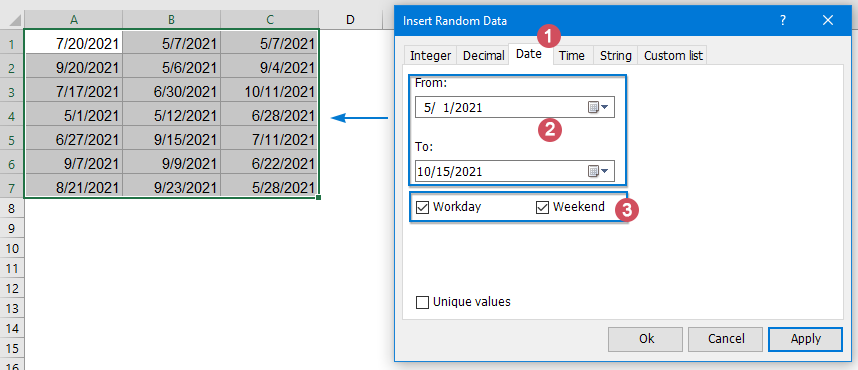Tips: To generate some random distinct dates, please check Unique values option.

#### 1.11 Generate random times in Excel

After inserting random numbers, text strings and dates, in this section, I will talk about some tricks for generating random times in Excel.

###### Generate random times with formulas

Generate random times with a formula

To generate random times in a range of cell, a formula based on the TEXT and RAND functions can do you a favor.

Please enter the below formula into a blank cell, and then drag and copy the formula to other cells where you want to get the times, see screenshot:

=TEXT(RAND(),"HH:MM:SS")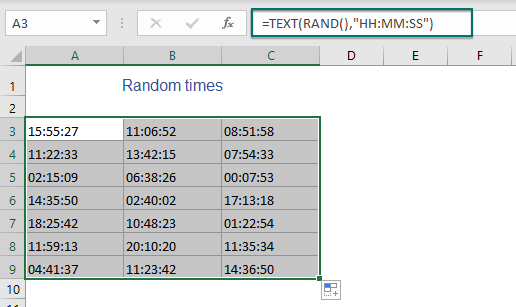Generate random times between two given times with a formula

If you need to insert some times randomly between two specific time, such as the times from 10 o’clock to 18 o’clock, please apply the below formula:

=TEXT(RAND()*(18-10)/24+10/24,”HH:MM:SS”)

Note: In the above formula, the number 18 is the end time, and 10 stands for the start time. You can change them to meet your demands.

And then, drag and copy the formula to other cells where you want to generate the random times between two given time ranges, see screenshot: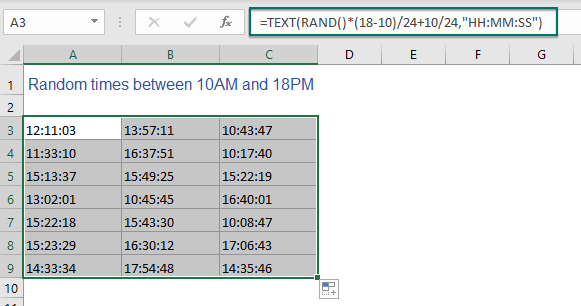Generate random times at specific intervals with a formula

Supposing, you want to come up with random times within specific intervals in Excel, such as inserting random times at a 15-minute interval. To deal with this job, you can use the RAND and FLOOR functions within the TEXT function.

Copy or enter the below formula into a blank cell, and then drag and copy this formula to the cells that you want to get the random times, see screenshot:

=TEXT(FLOOR(RAND(),"0:15"),"HH:MM:SS")

Note: In the formula, the number 15 is the time interval, if you need the random times at a 30-minute interval, just replace the 15 with 30.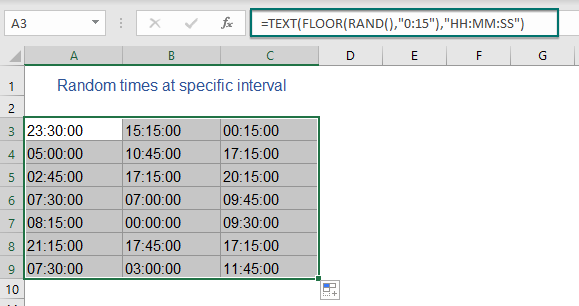###### Generate random times between two given times with a handy feature

If you have Kutools for Excel, its Insert Random Data feature also can help you to generate random times between given times in a worksheet.

After installing Kutools for Excel, please do as this:

1. Select a range of cells where to generate the times.

2. Then click Kutools > Insert > Insert Random Data, in the popped-out dialog box, please do the following operations:

• Click the Time tab;
• Then, specify the time range. In this example, I will choose from 9:00 AM to 16:30 PM.
• At last, click Ok or Apply to generate random times as below screenshot shown.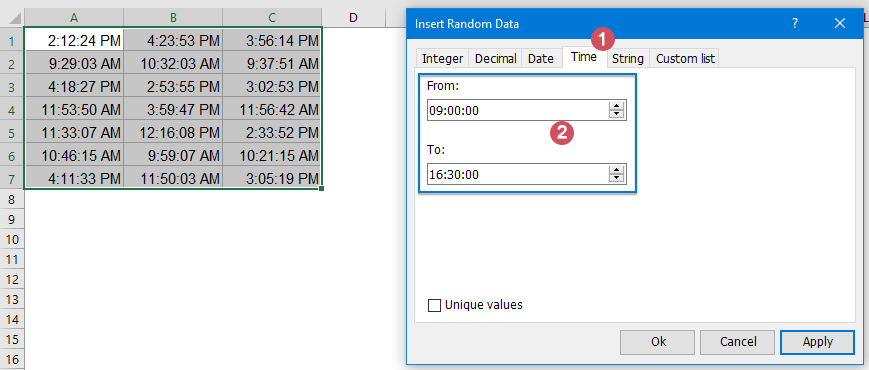###### Generate random dates and times between two datetimes with a formula

If you want to generate random dates and times together, the below formula can help you.

1. Enter or copy the following formula into a cell where you want to generate the random datetimes:

=TEXT(RAND()*("2021-10-15 12:00:00"-"2021-1-1 9:00")+"2021-1-1 9:00:00","YYYY-MM-DD HH:MM:SS")

Note: In this formula, 2021-10-15 12:00:00 is the end date and time, and 2021-1-1 9:00:00 is the start date and time, you can modify them to your need.

2. Then, drag and copy this formula to other cells where you want the random datetimes to be displayed, see screenshot:### Generate random numbers, texts, dates in Excel 365 / 2021

This section will show how to generate random numbers, dates, and get random selection and assign data to groups randomly in Excel 365 or Excel 2021 with a new dynamic array function - RANDARRAY.

The RANDARRAY function is used to return an array of random numbers between any two numbers that you specify.

The syntax of RANDARRAY function is:

=RANDARRAY([rows],[columns],[min],[max],[integer])
• rows (optional): The number of rows of random numbers to return; (If omitted, default =1)
• columns (optional): The number of columns of random numbers to return; (If omitted, default =1)
• min (optional): The minimum number to be returned; (If omitted, default =0)
• max (optional): The maximum number to be returned; (If omitted, default =1)
• integer (optional): Return a whole number or a decimal value. TRUE for a whole number, False for a decimal number. (If omitted, default =FALSE)
Notes:
• 1. There are five arguments in the RANDARRAY function, all of them are optional, if none of the arguments is specified, the RANDARRAY will return a decimal value between 0 and 1.
• 2. If the rows or columns arguments are decimal numbers, they will be truncated to the whole number before the decimal point (e.g. 3.9 will be treated as 3).
• 3. The minimum number must be less than the maximum number, otherwise it will return a #VALUE! error.
• 4. This RANDARRAY returns an array, when RANDARRAY returns multiple results in a worksheet, results will spill into adjacent cells.

#### 2.1 Generate random numbers in Excel 365 / 2021

To generate random whole or decimal numbers in Excel 365 or Excel 2021, you can use this new RANDARRAY function.

###### Generate random numbers between two numbers with a formula

To create a list of random numbers within a specific range, please apply the following formulas:

Please enter any of the below formulas as you need, and then press Enter key to get the results, see screenshots:

=RANDARRAY(6, 4, 50, 200, TRUE)               (Generate random integers between 50 and 200)
=RANDARRAY(6, 4, 50, 200, FALSE)
(Generate random decimals between 50 and 200)
Note: In the above formulas:
• 6: Indicates to return 6 rows of random numbers;
• 4: Indicates to return 4 columns of random numbers;
• 50, 200: The minimum and maximum values you want to generate numbers between;
• TRUE: Indicates to return whole numbers;
• FALSE: Indicates to return decimal numbers.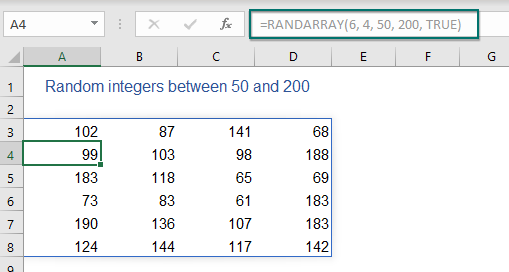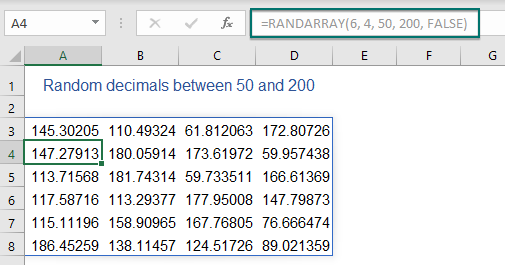###### Generate random numbers without duplicates with formulas

When using the normal RANDARRAY function to generate random numbers, some duplicate numbers will be created as well. To avoid the duplicates, here, I will talk about some formulas for solving this task.

Generate a list of non-repeating random numbers

To generate a column or a list of unique numbers randomly, the generic syntaxes are:

Random integers without duplicates:

=INDEX(UNIQUE(RANDARRAY(n^2, 1, min, max, TRUE)), SEQUENCE(n))

Random decimals without duplicates:

=INDEX(UNIQUE(RANDARRAY(n^2, 1, min, max, FALSE)), SEQUENCE(n))
• n: The number of values you want to generate;
• min: The minimum value;
• max: The maximum value.

For example, here, I will insert a list of 8 random numbers from 50 to 100 with no repeats, please apply the below any formulas you need, and then press Enter key to get the results:

=INDEX(UNIQUE(RANDARRAY(8^2, 1, 50, 100, TRUE)), SEQUENCE(8))          (Unique random integers)
=INDEX(UNIQUE(RANDARRAY(8^2, 1, 50, 100, FALSE)), SEQUENCE(8))
(Unique random decimals)
Note: In the above formulas:
• 8: Indicates to return 8 random numbers;
• 50, 100: The minimum and maximum value you want to generate numbers between.
• TRUE: Indicates to return whole numbers;
• FALSE: Indicates to return decimal numbers.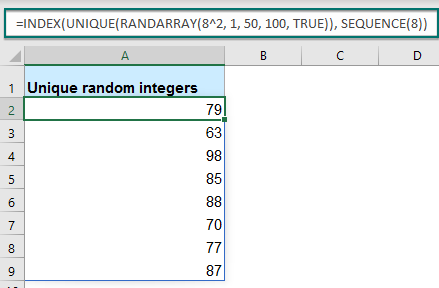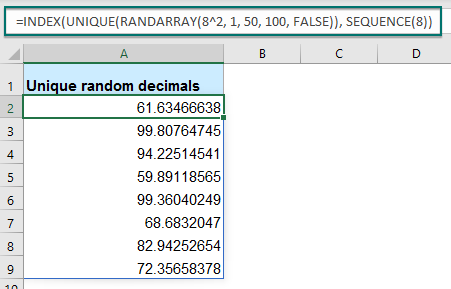Generate a range of non-repeating random numbers

If you want to generate non-repeating random numbers in a range of cells, you just need to define the number of rows and columns into the SEQUENCE function, the generic syntaxes are:

To generate a column or a list of unique numbers randomly, the generic syntaxes are:

Random integers without duplicates:

=INDEX(UNIQUE(RANDARRAY(n^2, 1, min, max, TRUE)), SEQUENCE(rows, columns))

Random decimals without duplicates:

=INDEX(UNIQUE(RANDARRAY(n^2, 1, min, max, FALSE)), SEQUENCE(rows, columns))
• n: The number of cells to insert the numbers, you can supply it as number of rows * number of columns; For example, to fill 8 rows and 3 columns, use 24^2.
• rows: The number of rows to fill;
• columns: The number of columns to fill;
• min: The lowest value;
• max: The highest value.

Here, I will fill a range of 8 rows and 3 columns with unique random numbers from 50 to 100, please apply any of the below formulas you need:

=INDEX(UNIQUE(RANDARRAY(24^2, 1, 50, 100, TRUE)), SEQUENCE(8,3))          (Unique random integers)
=INDEX(UNIQUE(RANDARRAY(24^2, 1, 50, 100, FALSE)), SEQUENCE(8,3))
(Unique random decimals)
Note: In the above formulas:
• 24: Indicates to return 24 random numbers, the product of 8 and 3 (rows*columns);
• 50, 100: The minimum and maximum values you want to generate numbers between;
• TRUE: Indicates to return whole numbers;
• FALSE: Indicates to return decimal numbers.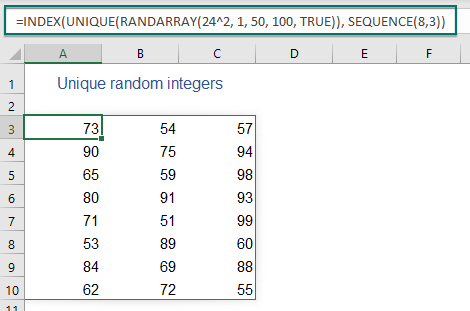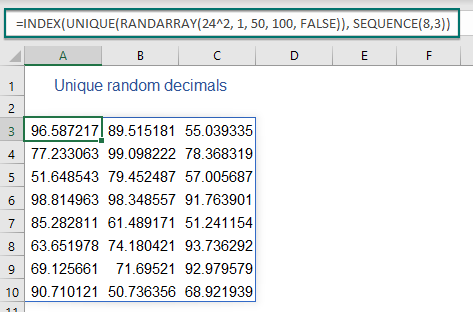#### 2.2 Generate random dates in Excel 365 / 2021

By using this new RANDARRAY function, you can also generate multiple random dates or workdays in Excel quickly and easily.

###### Generate random dates between two dates with a formula

To create a list of random dates between two specific dates, you can apply the below formula:

1. Enter the following formula into a blank cell to generate the random dates, and press Enter key to get a list of five-digit numbers, see screenshot:

=RANDARRAY(10, 1, B1, B2, TRUE)
Note: In the above formulas:
• 10: Indicates to return 10 rows of random dates;
• 1: Indicates to return 1 column of random dates;
• B1, B2: The cells contain the start and end dates that you want to generate dates between.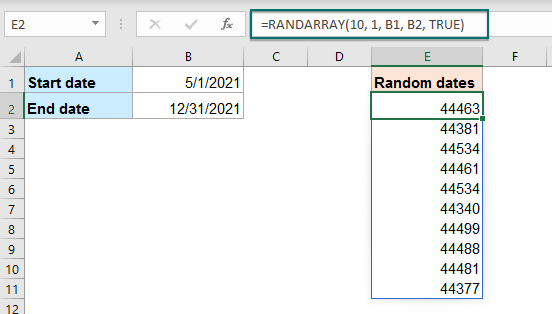2. Then, you should format the numbers to normal date format: Select the numbers, and then right click, then choose Format Cells from the context menu. in the following Format Cells dialog box, please do as this:

• Click Number tab;
• Then click Date from the Category pane;
• And then, choose one date formatting you like from the Type list box.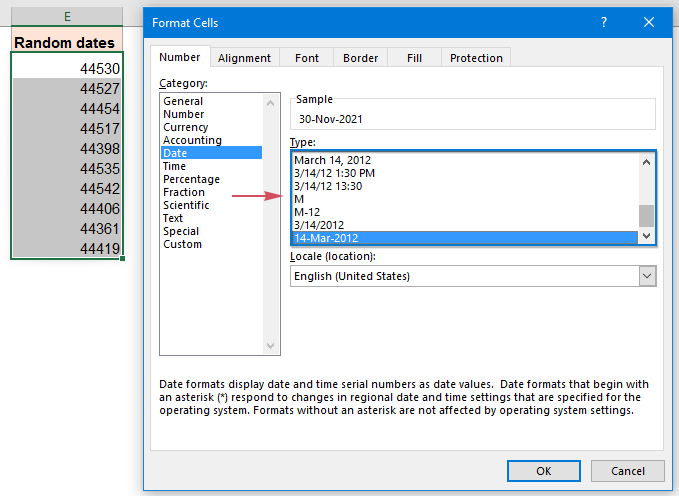3. And then, click OK button, the numbers will be formatted in the date format you specified, see screenshot: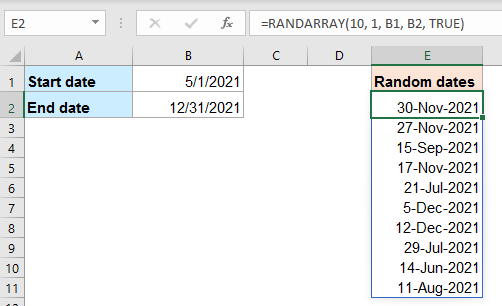Tips: Of course, you can also type the start date and end date directly into the formula as this:

=RANDARRAY(10, 1, "5/1/2021", "12/31/2021", TRUE)

###### Generate random workdays between two dates with a formula

To produce random workdays in a range of cells, you just need to embed the RANDARRAY function in the WORKDAY function.

1. Enter or copy the below formula into a blank cell, and then, press Enter key to get a list of numbers as below screenshot shown:

=WORKDAY(RANDARRAY(10, 1, B1, B2, TRUE), 1)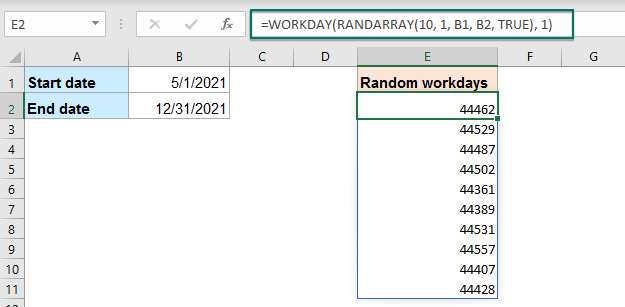2. Then, format the numbers to a specific date formatting as you need in the Format Cells dialog box, and you will get the normal date format as below screenshot shown: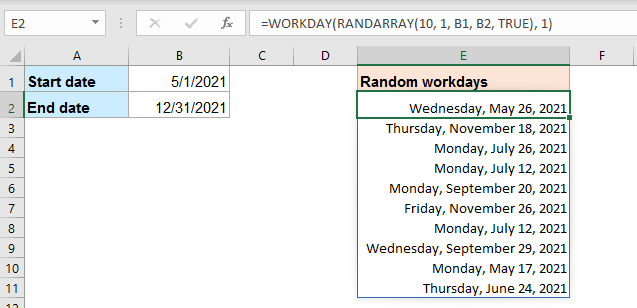Tips: You can also type the start date and end date into the formula directly as this:

=WORKDAY(RANDARRAY(10, 1, "5/1/2021", "12/31/2021", TRUE), 1)

#### 2.3 Generate or get random values from a list in Excel 365 / 2021

In Excel 365 or 2021, if you want to generate or return some random values from a list of cells, this section will introduce some formulas for you.

###### Generate or get random values from a list with a formula

To extract random values from a list of cells, this RANDARRY function with the INDEX function can do you a favor. The generic syntax is:

=INDEX(data, RANDARRAY(n, 1, 1, ROWS(data), TRUE))
• data: The list of values that you want to extract random items from;
• n: The number of random items you wish to extract.

For example, to extract 3 names from the name list A2:A12, please use the below formulas:

=INDEX(A2:A12, RANDARRAY(C2, 1, 1, ROWS(A2:A12), TRUE))             （Use a cell reference）
=INDEX(A2:A12, RANDARRAY(3, 1, 1, ROWS(A2:A12), TRUE))
(Type a number directly)

Then, press Enter key, and you will get 3 names randomly at once, see screenshot: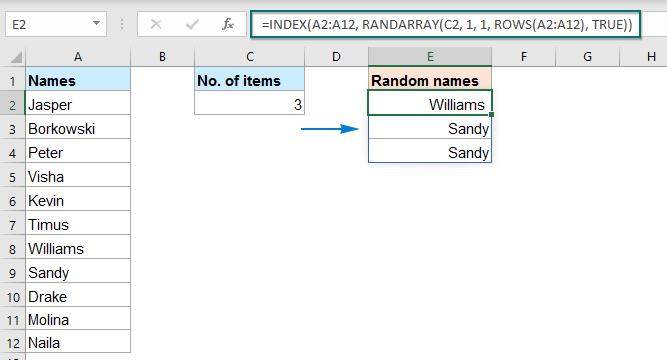###### Generate or get random values from a list without duplicates with a formula

With the above formula, you may find duplicates in the results. To make a random selection from a list with no repeats, the generic syntax is:

=INDEX(SORTBY(data, RANDARRAY(ROWS(data))), SEQUENCE(n))
• data: The list of values that you want to extract random items from;
• n: The number of random items you wish to extract.

If you need to return 5 names from the name list A2:A12 randomly, please enter or copy one of the below formulas:

=INDEX(SORTBY(A2:A12, RANDARRAY(ROWS(A2:A12))), SEQUENCE(C2))             (Use a cell reference)
=INDEX(SORTBY(A2:A12, RANDARRAY(ROWS(A2:A12))), SEQUENCE(5))
(Type a number directly)

Then, press Enter key to get 5 random names from the list A2:A12 with no repeats, see screenshot: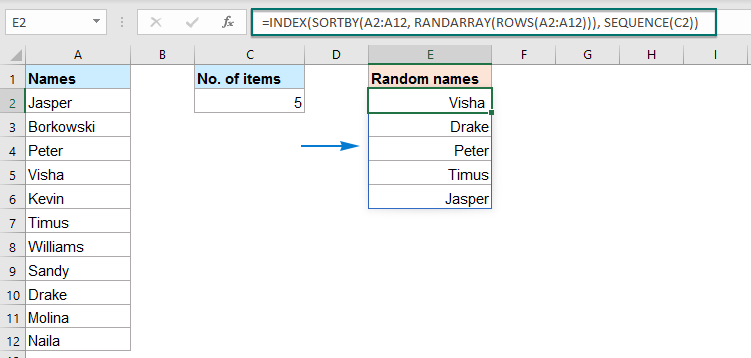#### 2.4 Generate or select random rows from a range in Excel 365 / 2021

Sometimes, you may need to pick up some random rows from a range of cells in Excel. To achieve this task, here, I will talk about some formulas.

###### Generate or select random rows from a range with a formula

The generic syntax to generate random rows from a range of cells is:

=INDEX(data, RANDARRAY(n, 1, 1, ROWS(data), TRUE), {1,2,3…})
• data: The range of cells that you want to extract random rows from;
• n: The number of random rows you wish to extract;
• {1,2,3…}: The column numbers to extract.

For extracting 3 rows of data from the range A2:C12, please use any of the following formulas:

=INDEX(A2:C12, RANDARRAY(E2, 1, 1, ROWS(A2:C12), TRUE), {1,2,3})               (Use a cell reference)
=INDEX(A2:C12, RANDARRAY(3, 1, 1, ROWS(A2:C12), TRUE), {1,2,3})
(Type a number directly)

Then, press Enter key to get 3 random rows of data from the range A2:C12, see screenshot: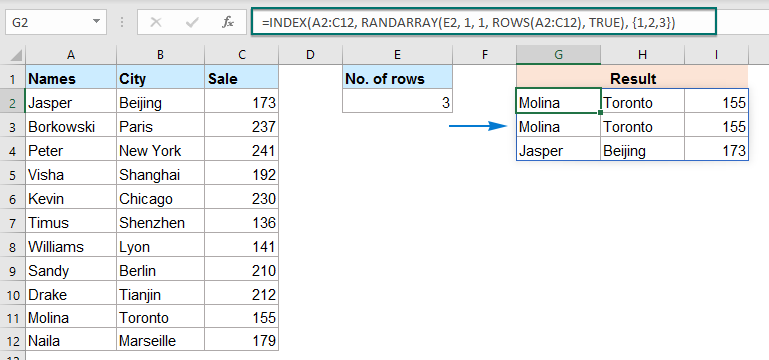###### Generate or select random rows from a range without duplicates with a formula

Similarly, the above formula can produce duplicate data as well. To prevent the duplicate rows from occurring, you can use the generic syntax below:

=INDEX(SORTBY(data, RANDARRAY(ROWS(data))), SEQUENCE(n), {1,2,3…})
• data: The range of cells that you want to extract random rows from;
• n: The number of random rows you wish to extract;
• {1,2,3…}: The column numbers to extract.

For instance, to pick up 5 rows of data from the range A2:C12, please use any of the below formulas:

=INDEX(SORTBY(A2:C12, RANDARRAY(ROWS(A2:C12))), SEQUENCE(E2), {1,2,3})            (Use a cell reference)
=INDEX(SORTBY(A2:C12, RANDARRAY(ROWS(A2:C12))), SEQUENCE(5), {1,2,3})
(Type a number directly)

And then, press Enter key, 5 random rows without duplicates will be extracted from range A2:C12 as below screenshot shown: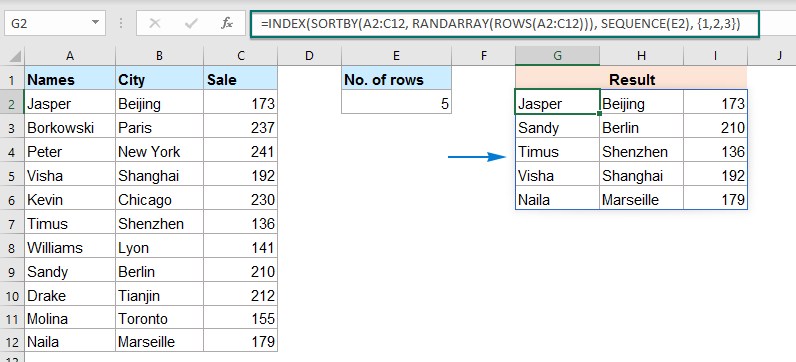### Prevent the random results from changing

Probably, you have noticed that all of the randomizing functions in this article, such as RAND, RANDBETWEEN and RANDARRAY are volatile. The generating results will be recalculated every time when the sheet is changed, and random new values will be produced afterwards. To stop the random values from changing automatically, here are two quick tricks for you.

###### Prevent the random results from changing by using copy and paste

Normally, you can apply the Copy and Paste feature to copy and paste the dynamic formulas as values, please do as this:

1. Select the cells with your random formula, and then press Ctrl + C to copy them.

2. Then, right click the selected range, and click Values option from the Paste Options section, see screenshot:

Tips: You can also press Shift + F10 and then V to activate this option.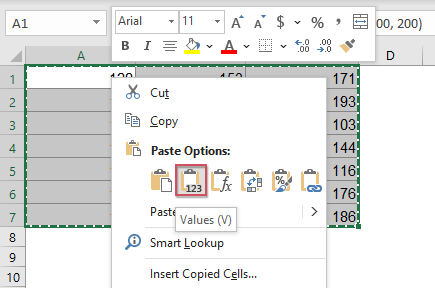3. And all the formula cells will be converted to values, the random values will not change any more.

###### Prevent the random results from changing by using a handy feature

If you have installed Kutools for Excel, the To Actual feature can help you to convert all selected formula cells to values with only one click.

After installing Kutools for Excel, please do as this:

1. Select the cells with the random formula, and then click Kutools > To Actual, see screenshot: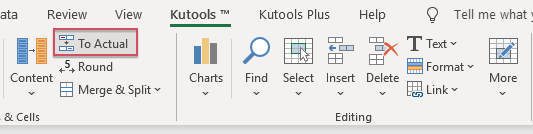2. And now, all the selected formulas have been converted to values.

## The Best Office Productivity Tools

### Kutools for Excel Solves Most of Your Problems, and Increases Your Productivity by 80%

• Super Formula Bar (easily edit multiple lines of text and formula); Reading Layout (easily read and edit large numbers of cells); Paste to Filtered Range...
• Merge Cells/Rows/Columns and Keeping Data; Split Cells Content; Combine Duplicate Rows and Sum/Average... Prevent Duplicate Cells; Compare Ranges...
• Select Duplicate or Unique Rows; Select Blank Rows (all cells are empty); Super Find and Fuzzy Find in Many Workbooks; Random Select...
• Exact Copy Multiple Cells without changing formula reference; Auto Create References to Multiple Sheets; Insert Bullets, Check Boxes and more...
• Favorite and Quickly Insert Formulas, Ranges, Charts and Pictures; Encrypt Cells with password; Create Mailing List and send emails...
• Extract Text, Add Text, Remove by Position, Remove Space; Create and Print Paging Subtotals; Convert Between Cells Content and Comments...
• Super Filter (save and apply filter schemes to other sheets); Advanced Sort by month/week/day, frequency and more; Special Filter by bold, italic...
• Combine Workbooks and WorkSheets; Merge Tables based on key columns; Split Data into Multiple Sheets; Batch Convert xls, xlsx and PDF...
• Pivot Table Grouping by week number, day of week and more... Show Unlocked, Locked Cells by different colors; Highlight Cells That Have Formula/Name...### Office Tab - brings tabbed interface to Office, and make your work much easier

• Enable tabbed editing and reading in Word, Excel, PowerPoint, Publisher, Access, Visio and Project.
• Open and create multiple documents in new tabs of the same window, rather than in new windows.
• Increases your productivity by 50%, and reduces hundreds of mouse clicks for you every day!